# Olympiad Test: Direct And Inverse Variations-2

## 10 Questions MCQ Test Mathematical Olympiad Class 8 | Olympiad Test: Direct And Inverse Variations-2

Description
Attempt Olympiad Test: Direct And Inverse Variations-2 | 10 questions in 20 minutes | Mock test for Class 8 preparation | Free important questions MCQ to study Mathematical Olympiad Class 8 for Class 8 Exam | Download free PDF with solutions
QUESTION: 1

### If 6 men can do a job in 8 days. In how many days can 8 men do it?

Solution:

If x is required days then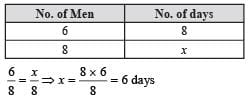QUESTION: 2

### A factory requires 42 machines to produce a given number of articles in 56 days. How many machines would be required to produce the same number of articles in 48 days?

Solution:

If x required machines then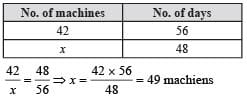QUESTION: 3

### A photograph of a bacteria enlarged 70000 times attains a length of 7 cm. What is the actual length of the bacteria?

Solution:

Here actual length of bacteria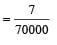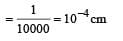QUESTION: 4

If 5 men or 7 women can earn ₹ 1372 per day. How much would 10 men and 5 women earn per day?

Solution:

Given 5 men = 7 women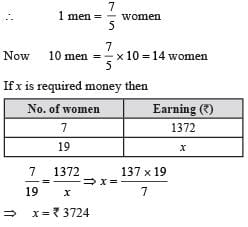QUESTION: 5

11 persons can dig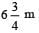long trench in one day. How many men should be employed for digging 27 m trench of the same type in one day?

Solution:

If x is required men then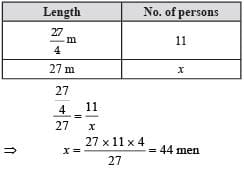QUESTION: 6

The scale of a map is 1:3 × 107. Two cities are 5cm apart on the map. What is the actual distance between them in kilometer ?

Solution:

If x is actual distance then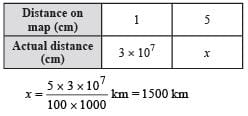QUESTION: 7

A loaded truck covers 18 km in 35 minutes. At the same speed how for can it travel in 7 hours?

Solution:

If x is required distance then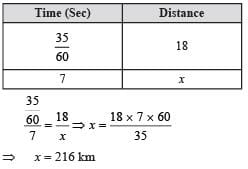QUESTION: 8

6 cows can graze a field in 28 days. How long would 21 cows take to graze the same field?

Solution:

If x is required no. of days then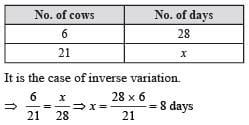QUESTION: 9

A car is travelling at a uniform speed of 84 km/hr. How much distance will it cover in 15 minutes?

Solution: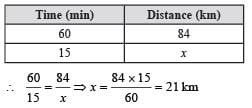QUESTION: 10

Ranjna types 510 words in half an hour. How many words would she type in 10 minutes?

Solution:

If x is required words then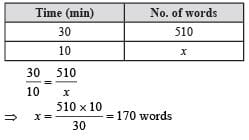Use Code STAYHOME200 and get INR 200 additional OFF Use Coupon Code